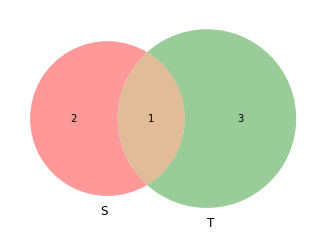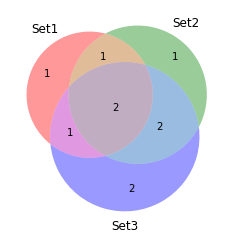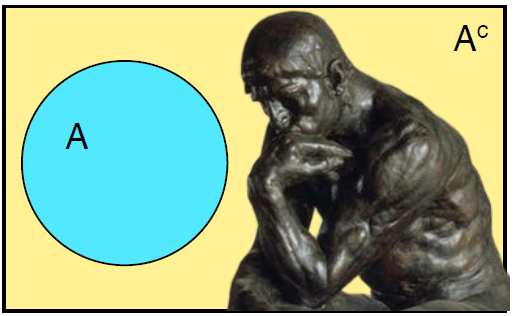# Set algebra with Python scripts¶

Set theory is a branch of mathematical logic that studies sets, which informally are collections of objects. Although any type of object can be collected into a set, set theory is applied most often to objects that are relevant to mathematics. The language of set theory can be used in the definitions of nearly all mathematical objects.

Set theory is commonly employed as a foundational system for modern mathematics, particularly in the form of Zermelo–Fraenkel set theory with the axiom of choice.

Python offers a native data structure called set, which can be used as a proxy for a mathematical set for almost all purposes.

In :
import IPython.display as disp


## Various ways to create a 'set' object in Python¶

In :
# Directly with curly braces
Set1 = {1,2}
print (Set1)

{1, 2}

In :
type(Set1)

Out:
set
In :
# By calling the 'set' function i.e. typecasting
Set2 = set({2,3})
print(Set2)

{2, 3}

In :
my_list=[1,2,3,4]
my_set_from_list = set(my_list)
print(my_set_from_list)

{1, 2, 3, 4}


Empty (Null) set is a special set $$\forall x, x \notin \varnothing$$

#### Do not try to create the empty set by declaring an empty {}. That denotes an empty dictionary object¶

In :
my_set = {}
print(type(my_set))

<class 'dict'>


#### Instead, use the set() function to create the empty (null) set from any empty data type e.g. dictionary or list¶

In :
my_set = set({})
print(type(my_set))
my_set_2 = set([])
print(type(my_set_2))

<class 'set'>
<class 'set'>


## Membership and size testing¶

### Membership testing by 'in' and 'not in' keywords¶

In :
my_set = set([1,3,5])
print("Here is my set:",my_set)
print("1 is in the set:",1 in my_set)
print("2 is in the set:",2 in my_set)
print("4 is NOT in the set:",4 not in my_set)

Here is my set: {1, 3, 5}
1 is in the set: True
2 is in the set: False
4 is NOT in the set: True


### Size checking by 'len' or 'not'¶

In :
S = {1,2}
not S

Out:
False
In :
T = set()
not T

Out:
True
In :
print("Size of S:", len(S))
print("Size of T:", len(T))

Size of S: 2
Size of T: 0


## Venn diagrams¶

In :
import matplotlib.pyplot as plt
import matplotlib_venn as venn
S = {1, 2, 3}
T = {0, 2, -1, 5}
venn.venn2([S, T], set_labels=('S','T'))
plt.show()In :
venn.venn3(subsets = (1, 1, 1, 2, 1, 2, 2), set_labels = ('Set1', 'Set2', 'Set3'))
plt.show()## Set relations¶

• Subset
• Superset
• Disjoint
• Universal set
• Null set
In :
Univ = set([x for x in range(11)])
Super = set([x for x in range(11) if x%2==0])
disj = set([x for x in range(11) if x%2==1])
Sub = set([4,6])
Null = set([x for x in range(11) if x>10])

In :
print("Universal set (all the positive integers up to 10):",Univ)
print("All the even positive integers up to 10:",Super)
print("All the odd positive integers up to 10:",disj)
print("Set of 2 elements, 4 and 6:",Sub)
print("A null set:", Null)

Universal set (all the positive integers up to 10): {0, 1, 2, 3, 4, 5, 6, 7, 8, 9, 10}
All the even positive integers up to 10: {0, 2, 4, 6, 8, 10}
All the odd positive integers up to 10: {1, 3, 5, 7, 9}
Set of 2 elements, 4 and 6: {4, 6}
A null set: set()

In :
Super.issuperset(Sub)

Out:
True
In :
Super.issubset(Univ)

Out:
True
In :
Sub.issubset(Super)

Out:
True
In :
Sub.issuperset(Super)

Out:
False
In :
Super.isdisjoint(disj)

Out:
True

Alternatively, operators like '<=' or '>' can be used to check relations

In :
Super > Sub

Out:
True
In :
Sub <= Super

Out:
True

### Algebra of inclusion¶

If A, B and C are sets then the following hold:

Reflexivity:

$$A\subseteq A}$$

Antisymmetry:

$$A\subseteq B\ \ and\ B\subseteq A\ \text if\ and\ only\ if\ A=B$$

Transitivity:

$$If\ A\subseteq B}\ and \ B\subseteq C}, then\ A ⊆ C$$

## Set algebra/Operations¶

• Equality
• Intersection
• Union
• Complement
• Difference
• Cartesian product

### Equality/Non-equality of sets¶

In :
S1 = {1,2}
S2 = {2,2,1,1,2}
print ("S1 and S2 are equal because order or repetition of elements do not matter for sets\nS1==S2:", S1==S2)

S1 and S2 are equal because order or repetition of elements do not matter for sets
S1==S2: True

In :
S1 = {1,2,3,4,5,6}
S2 = {1,2,3,4,0,6}
print ("S1 and S2 are NOT equal because at least one element is different\nS1==S2:", S1==S2)

S1 and S2 are NOT equal because at least one element is different
S1==S2: False


### Intersection between sets¶

In mathematics, the intersection A ∩ B of two sets A and B is the set that contains all elements of A that also belong to B (or equivalently, all elements of B that also belong to A), but no other elements. Formally,

$$A\cap B=\{x:x\in A{\text{ and }}x\in B\}.}$$In :
# Define a set using list comprehension
S1 = set([x for x in range(1,11) if x%3==0])
print("S1:", S1)

S1: {9, 3, 6}

In :
S2 = set([x for x in range(1,5)])
print("S2:", S2)

S2: {1, 2, 3, 4}

In :
# Both intersection method or & can be used
S_intersection = S1.intersection(S2)
print("Intersection of S1 and S2:", S_intersection)

S_intersection = S1 & S2
print("Intersection of S1 and S2:", S_intersection)

Intersection of S1 and S2: {3}
Intersection of S1 and S2: {3}


One can chain the methods to get intersection with more than 2 sets

In :
S3 = set([x for x in range(4,10)])
print("S3:", S3)

S3: {4, 5, 6, 7, 8, 9}

In :
S1_S2_S3 = S1.intersection(S2).intersection(S3)
print("Intersection of S1, S2, and S3:", S1_S2_S3)

Intersection of S1, S2, and S3: set()


Now change the S3 to contain 3

In :
S3 = set([x for x in range(3,10)])
print("S3:", S3)
S1_S2_S3 = S1.intersection(S2).intersection(S3)
print("Intersection of S1, S2, and S3:", S1_S2_S3)

S3: {3, 4, 5, 6, 7, 8, 9}
Intersection of S1, S2, and S3: {3}


#### The symbol '&' can be used for intersection¶

In :
A = {1, 2, 3}
B = {5,3,1}
print("Intersection of {} and {} is: {} with size {}".format(A,B,A&B,len(A&B)))

Intersection of {1, 2, 3} and {1, 3, 5} is: {1, 3} with size 2Commutative law: $$A\cap B=B\cap A}$$

Associative law: $$(A\cap B)\cap C=A\cap (B\cap C)}$$

Distributive law: $$A\cap (B\cup C)=(A\cap B)\cup (A\cap C)}$$

### Union of sets¶

In set theory, the union (denoted by ∪) of a collection of sets is the set of all elements in the collection. It is one of the fundamental operations through which sets can be combined and related to each other. Formally,

$$A\cup B=\{x:x\in A{\text{ or }}x\in B\}$$
In :
# Both union method or | can be used
S1 = set([x for x in range(1,11) if x%3==0])
print("S1:", S1)
S2 = set([x for x in range(1,5)])
print("S2:", S2)

S_union = S1.union(S2)
print("Union of S1 and S2:", S_union)
S_union = S1 | S2
print("Union of S1 and S2:", S_union)

S1: {9, 3, 6}
S2: {1, 2, 3, 4}
Union of S1 and S2: {1, 2, 3, 4, 6, 9}
Union of S1 and S2: {1, 2, 3, 4, 6, 9}

In :
S3 = set([5*x for x in range(1,3)])
print("S3:", S3)
S4 = set ([7,8])
print("S4:", S4)
S1_S2_S3_S4 = S1.union(S2).union(S3).union(S4)
print("Union of S1, S2, S3, and S4:", S1_S2_S3_S4)

S3: {10, 5}
S4: {8, 7}
Union of S1, S2, S3, and S4: {1, 2, 3, 4, 5, 6, 7, 8, 9, 10}


#### The symbol '|' can be used for union¶

In :
A = {1, 2, 3}
B = {5,3,4}
print("Union of {} and {} is: {} with size {}".format(A,B,A|B,len(A|B)))

Union of {1, 2, 3} and {3, 4, 5} is: {1, 2, 3, 4, 5} with size 5We can prove the following identities (associative and distributive properties)

$$A\cup (B\cup C)=(A\cup B)\cup C}$$$$A\cap (B\cup C)=(A\cap B)\cup (A\cap C)}$$$$A\cup (B\cap C)=(A\cup B)\cap (A\cup C)}$$

### More algebra of inclusion involving union and intersection¶

If A, B and C are subsets of a set S then the following hold:

Existence of a least element and a greatest element:

$$\varnothing \subseteq A\subseteq S}$$

Existence of joins:

$$A\subseteq A\cup B}$$$$If\ A\subseteq C}\ and\ B\subseteq C,}\ then\ A\cup B\subseteq C}$$

Existence of meets: $$A\cap B\subseteq A}$$

$$If\ C\subseteq A}\ and\ C\subseteq B,}\ then\ C\subseteq A\cap B}$$

### Complement of set¶

In :
disp.Image("A-complement.PNG")

Out:If A is a set, then the absolute complement of A (or simply the complement of A) is the set of elements not in A. In other words, if U is the universe that contains all the elements under study, and there is no need to mention it because it is obvious and unique, then the absolute complement of A is the relative complement of A in U. Formally,

$$A^{\complement }=\{x\in U\mid x\notin A\}.}$$
In :
S=set([x for x in range (101) if x%2==0])
print ("S is the set of even numbers between 0 and 100:", S)

S is the set of even numbers between 0 and 100: {0, 2, 4, 6, 8, 10, 12, 14, 16, 18, 20, 22, 24, 26, 28, 30, 32, 34, 36, 38, 40, 42, 44, 46, 48, 50, 52, 54, 56, 58, 60, 62, 64, 66, 68, 70, 72, 74, 76, 78, 80, 82, 84, 86, 88, 90, 92, 94, 96, 98, 100}

In :
S_complement = set([x for x in range (101) if x%2!=0])
print ("S_complement is the set of odd numbers between 0 and 100:", S_complement)

S_complement is the set of odd numbers between 0 and 100: {1, 3, 5, 7, 9, 11, 13, 15, 17, 19, 21, 23, 25, 27, 29, 31, 33, 35, 37, 39, 41, 43, 45, 47, 49, 51, 53, 55, 57, 59, 61, 63, 65, 67, 69, 71, 73, 75, 77, 79, 81, 83, 85, 87, 89, 91, 93, 95, 97, 99}


You can take the union of two sets and if that is equal to the universal set (in the context of your problem), then you have found the right complement

In :
print ("Is the union of S and S_complement equal to all numbers between 0 and 100?",
S.union(S_complement)==set([x for x in range (101)]))

Is the union of S and S_complement equal to all numbers between 0 and 100? True


De Morgan's laws:

$$\left(A\cup B\right)^{\complement }=A^{\complement }\cap B^{\complement }.}$$$$\left(A\cap B\right)^{\complement }=A^{\complement }\cup B^{\complement }.}$$

Complement laws

$$A\cup A^{\complement }=U.}$$$$A\cap A^{\complement }=\varnothing .}$$$$\varnothing ^{\complement }=U.}$$$$U^{\complement }=\varnothing .}$$$${\text{If }}A\subset B{\text{, then }}B^{\complement }\subset A^{\complement }.}$$

### Just an example of De Morgan's laws¶

In :
A={-6,3,4,5}
B={-6,5,13}
U=A|B|{12,-2,-4}
print("U:",U)

U: {-4, 3, 4, 5, -6, 12, 13, -2}

In :
def complement_of_union(S1,S2,S3):
Su = S1|S2
S4 = set()
for item in S3:
if item not in Su:
return S4

def intersection_of_complement(S1,S2,S3):
S1C = set()
S2C = set()
for item in S3:
if item not in S1:
for item in S3:
if item not in S2:
return (S1C & S2C)

In :
complement_of_union(A,B,U) == intersection_of_complement(A,B,U)

Out:
True
In :
complement_of_union(A,B,U)

Out:
{-4, -2, 12}
In :
intersection_of_complement(A,B,U)

Out:
{-4, -2, 12}

### Difference between sets¶

If A and B are sets, then the relative complement of A in B, also termed the set-theoretic difference of B and A, is the set of elements in B but not in A.

$$B\setminus A=\{x\in B\mid x\notin A\}.}$$In :
S1 = set([x for x in range(31) if x%3==0])
print ("Set S1:", S1)

Set S1: {0, 3, 6, 9, 12, 15, 18, 21, 24, 27, 30}

In :
S2 = set([x for x in range(31) if x%5==0])
print ("Set S2:", S2)

Set S2: {0, 5, 10, 15, 20, 25, 30}

In :
S_difference = S2-S1
print("Difference of S1 and S2 i.e. S2\S1:", S_difference)

S_difference = S1.difference(S2)
print("Difference of S2 and S1 i.e. S1\S2:", S_difference)

Difference of S1 and S2 i.e. S2\S1: {25, 10, 20, 5}
Difference of S2 and S1 i.e. S1\S2: {3, 6, 9, 12, 18, 21, 24, 27}


Following identities can be obtained with algebraic manipulation:

$$C\setminus (A\cap B)=(C\setminus A)\cup (C\setminus B)}$$$$C\setminus (A\cup B)=(C\setminus A)\cap (C\setminus B)}$$$$C\setminus (B\setminus A)=(C\cap A)\cup (C\setminus B)}$$$$C\setminus (C\setminus A)=(C\cap A)}$$$$(B\setminus A)\cap C=(B\cap C)\setminus A=B\cap (C\setminus A)}$$$$(B\setminus A)\cup C=(B\cup C)\setminus (A\setminus C)}$$


$$A\setminus A=\emptyset}$$$$\emptyset \setminus A=\emptyset }$$$$A\setminus \emptyset =A}$$$$A\setminus U=\emptyset }$$

### Symmetric difference¶

In set theory, the symmetric difference, also known as the disjunctive union, of two sets is the set of elements which are in either of the sets and not in their intersection. $$A\,\triangle \,B=\{x:(x\in A)\oplus (x\in B)\}$$

$$A\,\triangle \,B=(A\smallsetminus B)\cup (B\smallsetminus A)}$$$$A\,\triangle \,B=(A\cup B)\smallsetminus (A\cap B)}$$Some properties,

$$A\,\triangle \,B=B\,\triangle \,A,}$$$$(A\,\triangle \,B)\,\triangle \,C=A\,\triangle \,(B\,\triangle \,C).}$$

The empty set is neutral, and every set is its own inverse:

$$A\,\triangle \,\varnothing =A,}$$$$A\,\triangle \,A=\varnothing .}$$
In :
print("S1",S1)
print("S2",S2)
print("Symmetric difference", S1^S2)
print("Symmetric difference", S2.symmetric_difference(S1))

S1 {0, 3, 6, 9, 12, 15, 18, 21, 24, 27, 30}
S2 {0, 5, 10, 15, 20, 25, 30}
Symmetric difference {3, 5, 6, 9, 10, 12, 18, 20, 21, 24, 25, 27}
Symmetric difference {3, 5, 6, 9, 10, 12, 18, 20, 21, 24, 25, 27}


### Cartesian Product¶

In set theory (and, usually, in other parts of mathematics), a Cartesian product is a mathematical operation that returns a set (or product set or simply product) from multiple sets. That is, for sets A and B, the Cartesian product A × B is the set of all ordered pairs (a, b) where a ∈ A and b ∈ B.

$$A\times B=\{\,(a,b)\mid a\in A\ {\mbox{ and }}\ b\in B\,\}.}$$

More generally, a Cartesian product of n sets, also known as an n-fold Cartesian product, can be represented by an array of n dimensions, where each element is an n-tuple. An ordered pair is a 2-tuple or couple. The Cartesian product is named after René Descartes whose formulation of analytic geometry gave rise to the concept

In :
A = set(['a','b','c'])
S = {1,2,3}

In :
def cartesian_product(S1,S2):
result = set()
for i in S1:
for j in S2:
return (result)

C = cartesian_product(A,S)
print("Cartesian product of A and S\n{} X {}:{}".format(A,S,C))

Cartesian product of A and S
{'a', 'c', 'b'} X {1, 2, 3}:{('b', 3), ('b', 2), ('a', 1), ('a', 2), ('a', 3), ('c', 3), ('c', 2), ('b', 1), ('c', 1)}

In :
print("Length of the Cartesian product set:",len(C))

Length of the Cartesian product set: 9


Note that because these are ordered pairs, same element can be repeated inside the pair i.e. even if two sets contain some identical elements, they can be paired up in the Cartesian product

In :
A = {1,2,3,4}
B = {2,3,4}

print ("Cartesian product of {} and {} is:\n{}".format(A,B,cartesian_product(A,B)))

Cartesian product of {1, 2, 3, 4} and {2, 3, 4} is:
{(1, 2), (3, 2), (1, 3), (3, 3), (4, 4), (1, 4), (2, 3), (4, 3), (2, 2), (4, 2), (3, 4), (2, 4)}


Instead of writing functions ourselves, we could use the itertools library of Python. Remember to turn the resulting product object into a list for viewing and subsequent processing

In :
from itertools import product as prod

In :
A = {1,2,3,4}
B = {2,3,4}
p=list(prod(A,B))
print (p)

[(1, 2), (1, 3), (1, 4), (2, 2), (2, 3), (2, 4), (3, 2), (3, 3), (3, 4), (4, 2), (4, 3), (4, 4)]


### Cartesian Power¶

The Cartesian square (or binary Cartesian product) of a set X is the Cartesian product $X^2 = X × X$. An example is the 2-dimensional plane $R^2 = R × R$ where R is the set of real numbers: $R^2$ is the set of all points (x,y) where x and y are real numbers (see the Cartesian coordinate system).

The cartesian power of a set X can be defined as:

$X^{n}=\underbrace {X\times X\times \cdots \times X} _{n}=\{(x_{1},\ldots ,x_{n})\ |\ x_{i}\in X{\text{ for all }}i=1,\ldots ,n\}.}$

The cardinality of a set is the number of elements of the set. Cardinality of a Cartesian power set is $|S|^{n}$ where |S| is the cardinality of the set S and n is the power.

We can easily use itertools again for calculating Cartesian power. The repeat parameter is used as power.

In :
A = {1,2,3} # 3 element set
p2=list(prod(A,repeat=2)) # Power set of power 2
print("Cartesian power 2 with length {}: {}".format(len(p2),p2))
print("\n")
p3=list(prod(A,repeat=3)) # Power set of power 3
print("Cartesian power 3 with length {}: {}".format(len(p3),p3))

Cartesian power 2 with length 9: [(1, 1), (1, 2), (1, 3), (2, 1), (2, 2), (2, 3), (3, 1), (3, 2), (3, 3)]

Cartesian power 3 with length 27: [(1, 1, 1), (1, 1, 2), (1, 1, 3), (1, 2, 1), (1, 2, 2), (1, 2, 3), (1, 3, 1), (1, 3, 2), (1, 3, 3), (2, 1, 1), (2, 1, 2), (2, 1, 3), (2, 2, 1), (2, 2, 2), (2, 2, 3), (2, 3, 1), (2, 3, 2), (2, 3, 3), (3, 1, 1), (3, 1, 2), (3, 1, 3), (3, 2, 1), (3, 2, 2), (3, 2, 3), (3, 3, 1), (3, 3, 2), (3, 3, 3)]

In :
A = {1, 2, 3}
k = 2
cartesian_power = set([i for i in prod(A, repeat = k)])
print("Tuples in %s^%i: " %(A,k))
for i in cartesian_power:
print(i)
print;
print("Size = ", len(cartesian_power))

Tuples in {1, 2, 3}^2:
(1, 2)
(3, 2)
(1, 3)
(3, 3)
(3, 1)
(2, 1)
(2, 3)
(2, 2)
(1, 1)
Size =  9